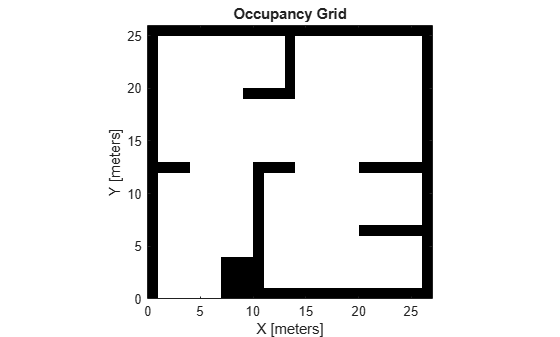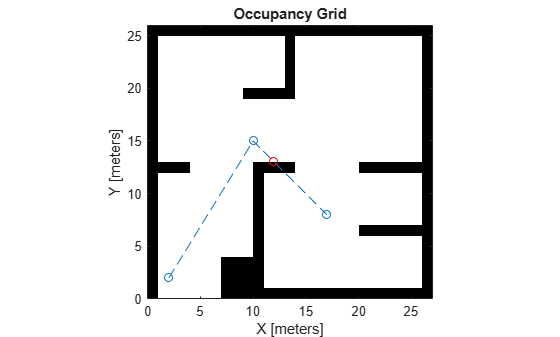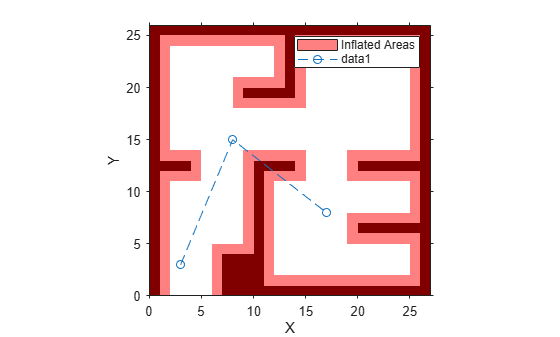# isMotionValid

Check if path between states is valid

## Syntax

``[isValid,lastValid] = isMotionValid(validator,state1,state2)``

## Description

example

````[isValid,lastValid] = isMotionValid(validator,state1,state2)` checks if the path between two states is valid by interpolating between states. The function also returns the last valid state along the path.```

## Examples

collapse all

This example shows how to validate paths through an evironment.

Load example maps. Use the simple map to create a binary occupancy map.

```load exampleMaps.mat map = occupancyMap(simpleMap); show(map)```Specify a coarse path through the map.

```path = [2 2 pi/2; 10 15 0; 17 8 -pi/2]; hold on plot(path(:,1),path(:,2),"--o")```Create a state validator using the `stateSpaceSE2` definition. Specify the map and the distance for interpolating and validating path segments.

```validator = validatorOccupancyMap(stateSpaceSE2); validator.Map = map; validator.ValidationDistance = 0.1;```

Check the points of the path are valid states. All three points are in free space, so are considered valid.

`isValid = isStateValid(validator,path)`
```isValid = 3x1 logical array 1 1 1 ```

Check the motion between each sequential path states. The `isMotionValid` function interpolates along the path between states. If a path segment is invalid, plot the last valid point along the path.

```startStates = [path(1,:);path(2,:)]; endStates = [path(2,:);path(3,:)]; for i = 1:2 [isPathValid, lastValid] = isMotionValid(validator,startStates(i,:),endStates(i,:)); if ~isPathValid plot(lastValid(1),lastValid(2),'or') end end hold off```This example shows how to validate paths through an evironment.

Load example maps. Use the simple map to create a vehicle cost map. Specify an inflation raidus of 1 meter.

```load exampleMaps.mat map = vehicleCostmap(double(simpleMap)); map.CollisionChecker = inflationCollisionChecker("InflationRadius",1); plot(map)```Specify a coarse path through the map.

```path = [3 3 pi/2; 8 15 0; 17 8 -pi/2]; hold on plot(path(:,1),path(:,2),"--o")```Create a state validator using the `stateSpaceSE2` definition. Specify the map and the distance for interpolating and validating path segments.

```validator = validatorVehicleCostmap(stateSpaceSE2); validator.Map = map; validator.ValidationDistance = 0.1;```

Check the points of the path are valid states. All three points are in free space, so are considered valid.

`isValid = isStateValid(validator,path)`
```isValid = 3x1 logical array 1 1 1 ```

Check the motion between each sequential path states. The `isMotionValid` function interpolates along the path between states. If a path segment is invalid, plot the last valid point along the path.

```startStates = [path(1,:);path(2,:)]; endStates = [path(2,:);path(3,:)]; for i = 1:2 [isPathValid, lastValid] = isMotionValid(validator,startStates(i,:),endStates(i,:)); if ~isPathValid plot(lastValid(1),lastValid(2),'or') end end hold off```This example shows how to validate paths through a 3-D occupancy map evironment.

Load and Assign Map to State Validator

Load the 3-D occupancy map of a city block. Specify the threshold to consider cells as obstacle-free.

```mapData = load('dMapCityBlock.mat'); omap = mapData.omap; omap.FreeThreshold = 0.5;```

Inflate the map to add a buffer zone for safe operation around the obstacles.

`inflate(omap,5);`

Create an SE(3) state space object with bounds for state variables.

`ss = stateSpaceSE3([-20 220;-20 220;-10 100; inf inf; inf inf; inf inf; inf inf]);`

Create an `occupancyMap3D` based state validator using the created state space.

`sv = validatorOccupancyMap3D(ss);`

Assign the map to the state validator object. Specify the `ValidationDistance` property.

```sv.Map = omap; sv.ValidationDistance = 0.1;```

Plan and Visualize Path

Create the path planner and increase the maximum connection distance. Reduce the maximum number of iterations.

```planner = plannerRRT(ss,sv); planner.MaxConnectionDistance = 50; planner.MaxIterations = 1000;```

Create a user-defined goal reached evaluation function. Specify the `GoalBias` property.

```planner.GoalReachedFcn = @(~,x,y)(norm(x(1:3)-y(1:3))<5); planner.GoalBias = 0.1;```

Set the start and goal states.

```start = [40 180 25 0.7 0.2 0 0.1]; goal = [150 33 35 0.3 0 0.1 0.6];```

Plan a path with default settings.

`[pthObj,solnInfo] = plan(planner,start,goal);`

Check the points of the path are valid states.

`isValid = isStateValid(sv,pthObj.States)`
```isValid = 6×1 logical array 1 1 1 1 1 1 ```

Check the motion between each sequential path states.

```isPathValid = zeros(size(pthObj.States,1)-1,1,'logical'); for i = 1:size(pthObj.States,1)-1 [isPathValid(i), ~] = isMotionValid(sv,pthObj.States(i,:),pthObj.States(i+1,:)); end isPathValid```
```isPathValid = 5×1 logical array 1 1 1 1 1 ```

Visualize the results.

```show(omap) hold on scatter3(start(1,1),start(1,2),start(1,3),'g','filled') % draw start state scatter3(goal(1,1),goal(1,2),goal(1,3),'r','filled') % draw goal state plot3(pthObj.States(:,1),pthObj.States(:,2),pthObj.States(:,3),'r-','LineWidth',2) % draw path```## Input Arguments

collapse all

State validator object, specified as an object of subclass of `nav.StateValidator`. These are the predefined state validator objects:

Initial state positions, specified as an n-element row vector or m-by-n matrix. n is the dimension of the state space specified in `validator`. m is the number of states to validate.

Data Types: `single` | `double`

Final state positions, specified as an n-element row vector or m-by-n matrix. n is the dimension of the state space specified in `validator`. m is the number of states to validate.

Data Types: `single` | `double`

## Output Arguments

collapse all

Valid states, returned as an m-element logical column vector.

Data Types: `logical`

Final valid state along each path, returned as an n-element row vector or m-by-n matrix. n is the dimension of the state space specified in the state space property in `validator`. m is the number of paths validated. Each row contains the final valid state along the associated path.

Data Types: `single` | `double`